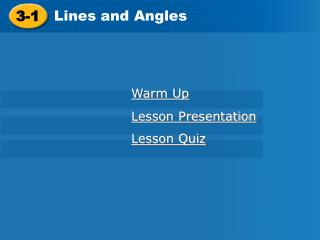Download Presentation3-1

# 3-1 - PowerPoint PPT PresentationDownload Presentation## 3-1

- - - - - - - - - - - - - - - - - - - - - - - - - - - E N D - - - - - - - - - - - - - - - - - - - - - - - - - - -
##### Presentation Transcript

1. 3-1 Lines and Angles Holt Geometry Warm Up Lesson Presentation Lesson Quiz

2. 3.1 Lines and Angles Warm Up Identify each of the following. 1.points that lie in the same plane 2. two angles whose sum is 180° 3. the intersection of two distinct intersecting lines 4. a pair of adjacent angles whose non-common sides are opposite rays coplanar points supplementary angles point linear pair

3. 3.1 Lines and Angles Objectives Identify parallel, perpendicular, and skew lines. Identify the angles formed by two lines and a transversal.

4. 3.1 Lines and Angles Vocabulary parallel lines perpendicular lines skew lines parallel planes transversal corresponding angles alternate interior angles alternate exterior angles same-side interior angles

5. 3.1 Lines and Angles

6. 3.1 Lines and Angles Helpful Hint Segments or rays are parallel, perpendicular, or skew if the lines that contain them are parallel, perpendicular, or skew.

7. 3.1 Lines and Angles LM ||QR KNand PQ NS SP Example 1: Identifying Types of Lines and Planes Identify each of the following. A. a pair of parallel segments B. a pair of skew segments C. a pair of perpendicular segments D. a pair of parallel planes plane NMR|| plane KLQ

8. 3.1 Lines and Angles BF || EJ BFand DE are skew. BF FJ Check It Out! Example 1 Identify each of the following. a. a pair of parallel segments b. a pair of skew segments c. a pair of perpendicular segments d. a pair of parallel planes plane FJH || plane BCD

9. 3.1 Lines and Angles

10. 3.1 Lines and Angles Example 2: Classifying Pairs of Angles Give an example of each angle pair. A. corresponding angles 1 and 5 B. alternate interior angles 3 and 5 C. alternate exterior angles 1 and 7 D. same-side interior angles 3 and 6

11. 3.1 Lines and Angles Check It Out! Example 2 Give an example of each angle pair. A. corresponding angles 1 and 3 B. alternate interior angles 2 and 7 C. alternate exterior angles 1 and 8 D. same-side interior angles 2 and 3

12. 3.1 Lines and Angles Helpful Hint To determine which line is the transversal for a given angle pair, locate the line that connects the vertices.

13. 3.1 Lines and Angles Example 3: Identifying Angle Pairs and Transversals Identify the transversal and classify each angle pair. A. 1 and 3 transversal l corr. s B. 2 and 6 transversal n alt. int s C. 4 and 6 transversal m alt. ext s

14. 3.1 Lines and Angles Check It Out! Example 3 Identify the transversal and classify the angle pair 2 and 5 in the diagram. transversal n same-side int. s.

15. 3.1 Lines and Angles EH || FG BFand EH CG GH Lesson Quiz: Part I Identify each of the following. 1. a pair of parallel segments 2. a pair of skew segments 3. a pair of perpendicular segments 4. a pair of parallel planes ABCand EFG

16. 3.1 Lines and Angles Lesson Quiz: Part II Identify each of the following. 5. one pairalternate interior angles EHG and HGK 6. One pair corresponding angles EHG and FGJ 7. one pairalternate exterior angles IHE and JGK 8. one pairsame-side interior angles EHG and HGF